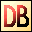DelphiBasicsSuccFunction Increment an ordinal variable System unit
 function Succ ( const Ordinal Value ) : Ordinal type;
Description
The Succ function increments an ordinal value, and returns this value.

You can increment :

 Characters Non-floating number types Enumeration types Pointers

The increment is by the base size of the unit. For example, incrementing a Pointer will be by 4 bytes if the pointer points to Words.

Notes
Succ is equivalent in performance to simple addition, or the Inc procedure.
Related commands
 Dec Decrement an ordinal variable Inc Increment an ordinal variable Pred Decrement an ordinal variable Sqr Gives the square of a number Sqrt Gives the square root of a number Sum Return the sum of an array of floating point valuesDownload this web site as a Windows program.